# RS Aggarwal Class 6 Solutions Chapter 21 - Concept Of Perimeter And Area Ex 21A(21.1)

## RS Aggarwal Class 6 Chapter 21 - Concept Of Perimeter And Area Ex 21A(21.1) Solutions Free PDF

The following figures are drawn on a sheet of squared paper. Count the number of squares enclosed by each figure and find its area, taking the area of each square as 1 cm2.

Q1: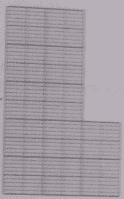Solution: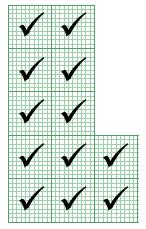The figure contains 12 complete squares.

Area of 1 small square = 1 sq. cm

Therefore, area of the figure = Number of complete squares × Area of the squares

= (12 × 1) sq cm

= 12 sq cm

Q2: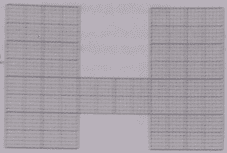Solution: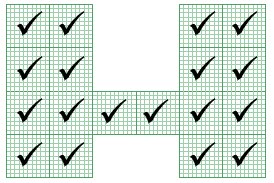The figure contains 18 complete squares.

Area of 1 small square = 1 sq. cm

Therefore, area of the figure = Number of complete squares × Area of the squares

= (18 × 1) sq cm

= 18 sq cm

Q3: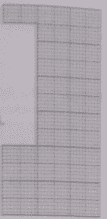Solution: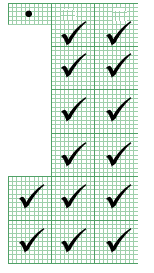The figure contains 14 complete squares and 1 half square.

Area of 1 small square = 1 sq. cm

Therefore, area of the figure = Number of complete squares × Area of the squares

= $left [ left ( 14 times 1 right ) + left ( 1 times frac{1} {2} right ) right ]; sq ; cm$

= $14frac {1} {2} sq ; cm$

Q4: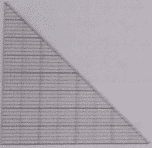Solution: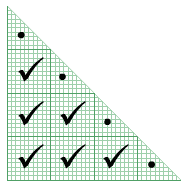The figure contains 6 complete squares and 4 half squares.

Area of 1 small square = 1 sq. cm

Therefore, area of the figure = Number of complete squares × Area of the squares

= $left [ left ( 6 times 1 right ) + left ( 4 times frac{1} {2} right ) right ]; sq ; cm$

= 8 sq cm

Q5.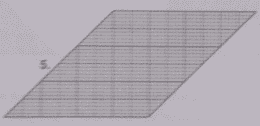Solution: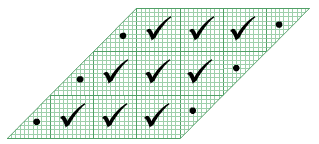The figure contains 9 complete squares and 6 half squares.

Area of 1 small square = 1 sq. cm

Therefore, area of the figure = Number of complete squares × Area of the squares

= $left [ left ( 9 times 1 right ) + left ( 6 times frac{1} {2} right ) right ]; sq ; cm$

= 12 sq cm

Q6.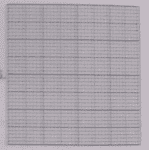Solution: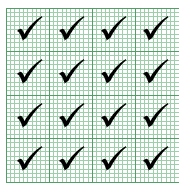The figure contains 16 complete squares.

Area of 1 small square = 1 sq. cm

Therefore, area of the figure = Number of complete squares × Area of the squares

= (16 × 1) sq cm

= 16 sq cm

Q7.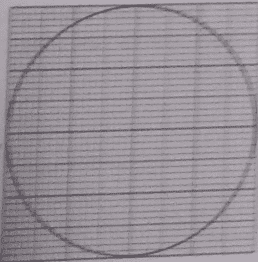Solution: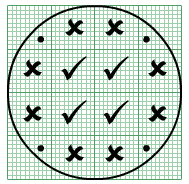In the given figure, there are 4 complete squares, 8 more than half parts of squares and 4 less than half parts of squares.

We neglect the less than half parts and consider each more than half part of the square as a complete square.

Therefore, Area = (4 + 8) sq cm = 12 sq cm

Q8.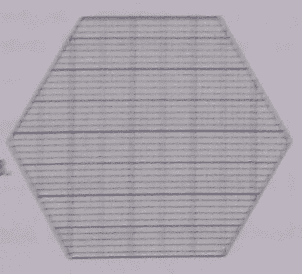Solution: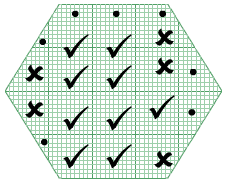In the given figure, there are 9 complete squares, 5 more than half parts of squares and 7 less than half parts of squares.

We neglect the less than half parts and consider each more than half part of the square as a complete square.

Therefore, Area = (9 + 5) sq cm = 14 sq cm

Q9.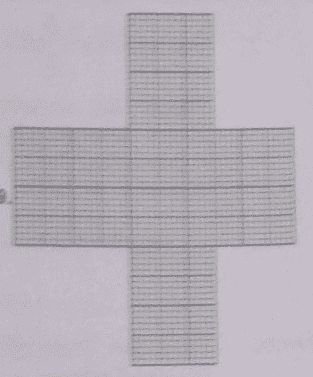Solution: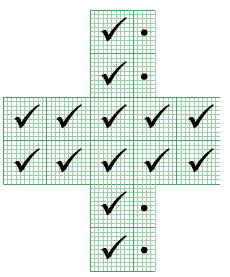The figure contains 14 complete squares and 4 half squares.

Area of 1 small square = 1 sq. cm

Therefore, area of the figure = Number of squares × Area of one squares

= $left [ left ( 14 times 1 right ) + left ( 4 times frac{1}{2} right ) right ]; sq ; cm$

= 16 sq cm

#### Practise This Question

Find the cost of putting grass beds on a pathway having area of 200 square meters at 0.1 paise per square cm.